# Rubik's nxnxn Cube Family

## Ken Fraser1

 Date of original document: 02 December 2010 Date of this revision: 17 February 2017

## Summary

While interest in Rubik's family cubes has dwindled since its 1980s peak when just about every household had one of these mechanical things to twiddle, there is still a strong body of interest among old and new stalwarts. Over time bigger hardware cubes and much bigger software cubes have become available. This document attempts to review the status of Rubik's family cubes into the 21st century.

The original version of this document with a minor change to reduce its size and small changes to make it Wikipedia compatible was placed on Wikipedia with title Rubik's family cubes of all sizes on 10 October 2012.

 1 Ken Fraser retired in 2002 as Principal Research Scientist and head of Helicopter Life Assessment at the Aeronautical and Maritime Research Laboratory (as it was known at the time), Defence Science and Technology Organisation, Department of Defence, Australia. This publication is the result of a leisure activity and has no relation to work at the Laboratory.

## Contents

1. Introduction

2. Definitions

3. Cube types

3.1 Hardware cubes

3.2 Software cubes

4. Cube design variants

4.1 Standard cubes with unmarked centres

4.2 Cubes with marked centres

5. Laws of Rubik's family cubes

6. Cube solving

6.1 Solving by people

6.2 Large cube issues

6.3 Solving by computer program

6.4 Final layer problems

6.5 Algorithms

6.6 Speedcubing

7. Reachable and unreachable states for cubes of all sizes

7.1 Relationship between reachable and unreachable states

7.2 Reachable states for cubes of all sizes

7.3 Unreachable states for cubes of all sizes

References

## 1. Introduction

The original Rubik's cube was a mechanical 3x3x3 cube puzzle invented in 1974 by the Hungarian sculptor and professor of architecture Erno Rubik. Extensions of the Rubik's cube have been around for a long time and come in both hardware and software forms. Major extensions have been the availability of cubes of larger size and the availability of the more complex cubes with marked centres. The properties of Rubik's family cubes of any size together with some special attention to software cubes is the main focus of this article. Many properties are functions of the cube size variable.

## 2. Definitions

 Cube size The standard Rubik's cube is often referred to as a 3x3x3 cube. That cube will be referred to as a size 3 cube and in general an n x n x n cube will be referred to as a size n cube. Rubik cube family Cubes that have similar rotational properties to the standard Rubik's size 3 cube and obey generalized rules for a size n cube are considered to be members of the Rubik cube family. Cubes of size 2 and above that meet this condition are available. Cubie Individual cube elements will be referred to as cubies (others sometimes refer to them as "cubelets"). There are three types of cubies: corner cubies (three coloured surfaces), edge cubies (two coloured surfaces) and centre cubies (one coloured surface). The absolute centre cubies for odd size cubes sit on the central axes of the six faces and their relative positions never change. Cubicle A cubicle is the compartment in which a cubie resides. For a permutation (defined below), cubicles are considered to occupy fixed positions relative to the cube object but their contents (cubies) may shift position. Facelet A facelet is a visible coloured surface of a cubie (corner cubies have three facelets, edge cubies have two and centre cubies have one). Cube state A particular arrangement of the cubies will be referred to as a cube state. What looks the same is considered to be the same (unless specific mention to the contrary is made). Each state has equal probability of being produced after a genuine random scrambling sequence. A rotation of the whole cube does not change the state considered herein. In other texts the various states are often referred to as permutations or arrangements. Cube layer A cube layer is a one cubie width slice of the cube perpendicular to its axis of rotation. Outer layers (faces) contain more cubies than inner layers. For a cube of size n there will be n layers along any given axis. Cube face The meaning of a cube face depends on the context in which it is used. It usually means one of the six three-dimensional outer layers but can also refer to just the outside layer's surface which is perpendicular to its axis of rotation. The faces are usually designated as up (U), down (D), front (F), back (B), left (L) and right (R). Set state The set (or solved) state of the cube is one for which a uniform colour appears on each of the six faces. For cubes with marked centres the set state also includes a unique arrangement of all centre cubies. Scrambled state The scrambled state is the starting point for unscrambling the cube. It arises when a cube in the set or any other state is subject to a large number of randomly chosen layer rotations. "Fixed-in-space" axes of rotation There are three mutually perpendicular axes of rotation for the cube. One set of axes defined in terms of D, U, B, F, L and R terms can be considered to have a fixed orientation in space. Think of these axes as belonging to a cube-shaped container in which the cube object can be positioned in any of 24 orientations. One axis can be drawn through the centres of the D and U faces (the DU axis). The others are the BF and LR axes. "Cube object" axes of rotation Another set of axes, can be defined for the cube object itself. These axes relate to the face colours, the most common being white, red, orange, yellow, green and blue. The axes are usually white-blue, red-orange and yellow-green. For odd size cubes these axes are always fixed relative to the internal frame of the cube object. For even size cubes these axes remain fixed relative to the internal frame of the cube object after initial selections. The origin for the axes is the centre of the cube object. Layer rotation The only way that cube state can be changed is by rotations of cube layers about their axes of rotation. All changes of state involve rotation steps that can be considered as a sequence of single layer quarter turns. Orbit For a basic quarter turn of a cube layer for cubes of all sizes, sets-of-four cubies move in separate four-cubicle trajectories. When all the possible trajectories for a given cubie type are considered for the whole cube we will refer to all the possible movement positions as being in a given orbit. We consider that the size 3 cube has two orbits, one in which the eight corner cubies are constrained to move and one in which the 12 edge cubies are constrained to move. Transfer of cubies between these orbits is impossible. For cubes of size 4 and above we will also define an edge cubie orbit as comprising 12 cubies but will use the term complementary orbit to describe a pair of orbits between which edge cubies can move. A pair of complementary edge cubie orbits contains a total of 24 cubies. Cubes of size 4 and above include centre cubie orbits that contain 24 cubies. Transfer of cubies between one such orbit and another is not possible (applies to cubes of size 5 and above). Move A move is a quarter turn rotation of a layer or a sequence of such quarter turns that a person would apply as a single step. Move notation A clockwise quarter turn of an outer layer is usually expressed as U, D, F, B, L or R. In other respects the notation used varies among authors. Algorithm An algorithm defines a sequence of layer rotations to transform a given state to another (usually less scrambled) state. Usually an algorithm is expressed as a printable character sequence according to some move notation. An algorithm can be considered to be a "smart" move. All algorithms are moves but few moves are considered to be algorithms. Permutation A permutation of the cube usually means the act of permuting (i.e. rearranging or changing) the positions of cubies. A permutation is an all-inclusive term which includes all moves (and algorithms) and more. Even the solving of the cube from a scrambled state represents a permutation. The term "permutation" is used extensively by mathematicians who use Group Theory to quantify the process involved in a rearrangement of cubies. The term "permutation" is also often used to mean the state of the cube that results after it is rearranged but that meaning will not be used herein. In such cases the term "cube state" will be used. That allows the term "permutation" to be used in situations where the permutation results in no change of state - a specific area of interest for permutations. Parity A cube permutation can be represented by a number of swaps of two cubies. If that number is even the permutation has even parity, and if the number is odd the permutation has odd parity.

Back to Contents.

## 3. Cube types

### 3.1 Hardware cubes

Hardware (physical) cubes are based on the original size 3 cube invented by Erno Rubik in 1974. These cubes usually use coloured stickers on the facelets for cubie identification. The size 3 (standard Rubik's cube) gained peak interest in the 1980s and was closely followed by the size 4 (Rubik's Revenge) cube. Other more recent well-known hardware forms of the cube come in the size 2 (Pocket Cube), the size 5 (Professor's Cube), the size 6 (V-Cube 6) and the size 7 (V-Cube 7). Less-known hardware cubes of larger sizes have also been produced.

### 3.2 Software cubes

In parallel with the hardware form of the cube there are many software forms available. Software cube emulators are not subject to the physical restraints that impose a size limit on the hardware forms. Hence the only really big cubes available are those in software form. Also, unlike the hardware forms, a range of cube sizes can easily be accommodated by the one program. The design characteristics of programs that allow users to unscramble cubes vary considerably with such features as the capability to allow the user to save a partially unscrambled state being often available.

Software cubes were in use in the 1980s when monochrome monitors were in common use. The lack of colour meant a different way of face identification was required. A program that retained 1980s monochrome capability (using numerals 1 to 6 to identify facelets) for cubes in the size 2 to 11 range was produced in1992 (together with colour capability in the size 2 to 15 range). More recently developed software cubes invariably use coloured facelets as for hardware cubes.

The most common, but by no means universal, approach is to emulate the cube by providing a "three-dimensional" display of the cube that makes it look like a real hardware cube. A drawback of the "three-dimensional" display is that, without some extra enhancements, the state of parts of the cube for any given view is hidden.

Other interactive software approaches that do not emulate a three-dimensional cube are also used by some programmers. Generally, an aim of such approaches is to allow the state of all cubies to be in view all the time but has the disadvantage (for some viewers) that the display does not appear like a real-world cube. A conventional two-dimensional (unfolded) display with all cube elements appearing of equal size is one approach. Another form of display where all cube elements do not appear of equal size is also in use. The upper cube size limit for software cubes is limited by the monitor's available pixels and what viewers find as acceptable which, in turn, is a function of their visual acuity. For cubes of large size it can be advantageous to allow a portion of the cube to be scrolled out of view.

All emulators provide a means for the user to change the cube state step-by-step and unscramble the cube. Most emulators use mouse movements to control the rotation of the cube elements, others use keyboard commands, and some use a combination of both.

Software cubes present some major capabilities that are not possible with hardware cubes. Instant return to the set state is always available. If the program allows a partially unscrambled state to be saved then, by regularly updating the saved state, users need not despair if they do something that leaves their cube in a mess. They can return to their previously recorded state and continue from there. The bigger the cube the more helpful such a capability becomes.

Some Freeware large cube (size greater than 10) implementations  are available.

Back to Contents.

## 4. Cube design variants

While there are multiple variants in use only two will be considered here:

• Standard cubes with unmarked centres.
• Cubes with marked centres.

Back to Contents.

### 4.1 Standard cubes with unmarked centres

A 2-layer (size 2) cube has corner cubies only.

Cubes of size 2 and size 3 have single solutions meaning that all the cube elements can have only one correct location for a solved cube.

Centre cubies differ from the corner and edge cubies in that their orientation or position has multiple possibilities. For cubes of odd size, there will be a centre cubie that is centrally located on the cube face and that cubie has only one correct location for a solved cube. However, multiple locations of all other centre cubies apply for a solved cube. The centre cubies (other than the single central one for cubes of odd size) form sets-of-four on each face and sets-of-24 for the whole cube for the various orbits. These centre cubies have four possible final positions (their orientation changes with position but cannot be changed independently) that would satisfy the solved state.

Back to Contents.

### 4.2 Cubes with marked centres

Typically, hardware cubes with marked centres use images or logos on the faces to designate what centre cubie(s) orientation is required for a solved cube. Application of such markings is generally restricted to just cubes of very small size.

Solving a cube with marked centres is considerably more complex than that for standard cubes. The use of jig-saw style image marking on cubes of large size would make a difficult task even more difficult. Two possibilities in current use on software cubes are the use of a numerical graphic in the "1" to "4" range and the use of a corner marking graphic.

There is a direct correspondence between numerical and corner marking. A top left corner quadrant marking is equivalent a numerical marking 1, second quadrant to 2, third quadrant to 3, and fourth quadrant to 4. The following table illustrates these different forms of marking.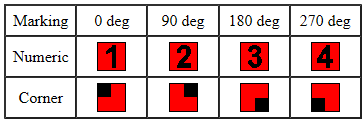Because transfer of cubies between orbits is impossible, the same 1-2-3-4 markings can be used for each orbit. With the exception of the absolute centre cubies for cubes of odd size, there are 24 centre cubies (4 per face) in each orbit. If n is cube size, there will be ((n - 2)2 - a)/4 orbits where a is zero if n is even or a is one if n is odd.

Numerical marking is typically applicable for cubes up to about size 32. Corner marking while less user-friendly can allow the marked centres range to be extended beyond the numerical marking limit.

Except for absolute centre marking for cubes of odd size, numerical marking would provide the best means of centre cubie marking for hardware cubes as their size range is limited. Rotation of the numbers would mean a small inconvenience relative to non-rotated numbers that can be used for software cubes. The big advantage of numbers is that they reduce the complexity of solving the last cube face when markings are in use (e.g. if the set-of-four sequence is 1-3-4-2 (even parity, needs two swaps to become the required 1-2-3-4) then the algorithm requirement is clear. Algorithms have been defined in and are, of course, equally applicable to hardware cubes.

Back to Contents.

## 5. Laws of Rubik's family cubes

A cube is solvable if the set state has existed some time in the past and if no tampering of the cube has occurred (e.g. by rearrangement of stickers on hardware cubes or by doing the equivalent on software cubes) has occurred. Laws for the standard size 3 Rubik's cube and for the complete Rubik's cube family have been documented. Those rules limit what arrangements are possible and mean that, of the possible unrestricted cubie arrangements, the number that are unreachable far outnumber those that are reachable.

Cubes of all sizes have three mutually perpendicular axes about which one or more layers can be rotated. All moves for the cube can be considered as a sequence of quarter-turn rotations about these axes. The movement possibilities give rise to a set of laws which in most cases can be expressed in analytical terms.

For a cube of size n:

 Number of corner cubies = 8 Number of edge cubies = 12(n - 2) Number of centre cubies = 6(n - 2)2 Number of facelets = 6n2 Total number of cubies = 6(n - 1)2 + 2 Increase in total number of cubies for unit increase in cube size from n to n + 1 = 12n - 6

Every cube move can be considered as a permutation. The relationship between the cube state after a move with that before a move can be expressed mathematically using Group Theory to quantify permutations. Since every move can be considered as a sequence of quarter turn rotations, it is appropriate to examine what is involved in a quarter turn rotation. Except for the absolute centre cubie for odd size cubes, during the quarter turn the cubies move in separate four-cubicle trajectories (also referred to as a 4-cycle movement since four quarter turns will restore the cubies in the specified trajectory to their original positions). A quarter turn of a 4-cubie set can be represented by three swaps as indicated below where swap 1-2 means the contents of cubicle 1 is swapped with the contents of cubicle 2, etc.

Start Swap 1-2 Swap 1-3 Swap 1-4
 1/A 2/B 4/D 3/C
 B A D C
 C A D B
 D A C B

The parity of a permutation refers to whether that permutation is even or odd. An even permutation is one that can be represented by an even number of swaps while an odd permutation is one that can be represented by an odd number of swaps. An odd permutation followed by an odd permutation will represent an overall even permutation (adding two odd numbers always returns an even number). Since a quarter turn is made up of a number of 4-cycles each involving three swaps, if the number of 4-cycles is odd, overall parity of the quarter turn permutation will be odd and vice versa.

Quarter turn permutation parity for a size n cube is given in the following table.

 Cube size (odd or even) Layer type Number of 4-cycle movements Overall parity odd inner n - 1 even odd outer ((n - 2)2 - 1)/4 + (n - 1) even* even inner n - 1 odd even outer (n/2 - 1)2 + (n - 1) even if n/2 is even **odd if n/2 is odd
 * Since ((n - 2)2 - 1) equals (n - 1)(n - 3), a product of two consecutive even numbers, which must always be evenly divisible by 8. ** Since (n/2 - 1)2 will be odd if (n/2 - 1) is odd (i.e. if n/2 is even) giving overall even since (n - 1) is odd. The reverse applies if n/2 is odd.

Summarising the above parity results we conclude:

• All permutations for odd size cubes have even overall parity.
• All individual quarter turns for even size cubes, where half the cube size is an odd number, have odd overall parity.
• For even size cubes where half the cube size is an even number, inner layer quarter turns have odd overall parity and outer layer quarter turns have even overall parity.

The above analysis considered the parity for corner (where applicable), edge and centre cubies combined. It is possible to consider these in isolation and when that is done an even combined quarter turn parity will involve a number of odd parity elements.

Standard cubes (i.e. cubes with unmarked centres) of any size greater than 3 behave exactly like the size 3 cube if only outer layer rotations are permitted. Parity rules dictate that, for cubes of odd size and for cubes of even size 6 and above, the swapping of the two cubies in a single edge set requires a change in the position of centre cubies. It can be shown that for the size 4 cube the swapping and inverting of the two complementary cubies in a single edge set can be achieved without any change in the position of any other cubies. It can also be shown that, for cubes of even size 6 and above, the swapping of the two cubies in a single edge set requires a change in the position of centre cubies

A permutation as used here takes account of the change in the positions of cubies, not any change in their orientations. For the 24 edge cubie sets (made up of 12 complementary pairs) for cubes of size greater than 3, there is no restriction on position. Orientation is set by position and cannot be changed independently of position.

Corner cubies behave the same for cubes of all sizes. They have three possible orientations made up from a combination of 1/3 twists where a full twist (about an axis drawn from the cube corner to the cubie's internal corner) returns the corner cubie to its original orientation. If we designate a unit clockwise twist by 1/3 and a unit counter-clockwise twist by -1/3, then the twist possibilities for a corner cubie relative to any given initial state (the set state for example) are 0, 1/3 and -1/3. The sum of the twist increments across all corner cubies must always be an integer (0, 1 or 2).

When inner layer rotations are included for cubes of size greater than 3, some of the edge cubie movement limitations mentioned above no longer apply. These are expanded on in the Final layer problems section.

Cubie position and orientation are of special concern when unscrambling the final layer. Edge cubies must always end up in exactly the same positions they occupied in the initial set state before scrambling. If any edge cubie in a given edge set in the final layer has a wrong orientation (only applicable to cubes of size greater than 3), it must be in a wrong position and will need to be swapped with a complementary edge cubie also having wrong orientation. With everything else in place, corner cubies can be in the right position but two or more can have incorrect orientation. For standard cubes of size greater than 3, centre cubies are extremely unlikely to occupy the same positions they had in the initial set state.

If permutations of facelets rather than cubies are considered then both position and orientation of cubies will be taken into account. For software cubes, the states (six colour possibilities) of the 6n2 facelets (in a 6 x n x n array for instance) is what would allow complete information on cube state to be saved for later use.

A cube of any size that is subject to repeats of the same permutation will eventually return to the state (e.g. the set state) it occupied before the first application of the permutation. The number of times that a permutation has to be applied to first return the cube to its initial state is referred to as the order or the cycle length of the permutation and is applicable to cubes of all sizes. The overall permutation that results in no change of state is referred to as an Identity Permutation. A program that allows permutation cycle length of any size cube to be determined is available and sample cycle length results have been documented. For a given permutation, cycle length can vary according to:

• Cube size.
• Initial cube state (for standard cubes with unmarked centres).
• Cube style (whether standard or marked centres are in use).
• Spatial orientation (checking all 24 of these rather than just one may give a different result).

The parity of an Identity Permutation is always even. That result for cubes of odd size is obviously true since every quarter turn has even parity. The result is less obvious for cubes of even size. For cubes of even size if the scrambling permutation relative to the previous set state is odd, then any permutation to solve the cube must also have odd parity and vice versa.

The generalized number of possible states for a size n cube is considered in the Reachable states for cubes of all sizes section.

Back to Contents.

## 6. Cube solving

### 6.1 Solving by people

Cube solving involves starting with a scrambled cube and applying step-by-step layer rotations to eventually end up with a solved cube. For cubes with unmarked centres that means all faces would need to appear in uniform colour. For cubes with marked centres a unique arrangement of all centre cubies in addition to the uniform colour requirement would need to apply. Since the starting point is always different, there can never be a unique set of rotations that can be applied to solve a cube. Usually, people work through the solution with the possible use of algorithms, mainly in the latter stage of the unscrambling. In theory, it is possible for a person to write a computer program that "thinks" like a human and solves the cube without human intervention (refer to the Solving by computer program section).

The aim of most software cube emulators is to provide a means for the user to interact with the program to solve (unscramble) the cube in a similar manner to the way they would unscramble a hardware cube.

Efficient rotation sequences (algorithms) can be developed using Group Theory permutation mathematics. However, there are many references to the relevant rotation sequences required to solve cubes of small size (refer to some for size 3, 4, and 5 cubes ) and there are multiple approaches to what steps that can be used. There is no such thing as a wrong way to solve a cube. The steps involved in solving any cube of size greater than 4 are fairly straight forward extensions of those required to solve size 3 and size 4 cubes. However, there is limited availability of generalized instructions that can be applied for solving cubes of any size (particularly the large ones). Generalized guidance on one way of solving standard cubes and cubes with marked centres of all sizes are available.

Anybody who can solve a size 4 cube should be able to solve cubes of larger size provided they accept an increased time-to-solve penalty.

In qualitative terms the complexity (difficulty) of solving a Rubik's family cube increases if the number of reachable states increases. Three main properties affect that number:

1. Cube size: The number of cubies to be placed is a quadratic (second order polynomial) function of cube size and therefore has a major influence on cube solving complexity.
2. Odd or even size: Even size cubes have an additional effect to just cube size that adds complexity relative to odd size cubes. This effect is relatively small and is independent of cube size (the added contribution when cube size changes from n to (n + 1) for n odd is constant). This effect will be expanded upon when the number of reachable states is considered later.
3. Unmarked or marked centre cubies: Centre cubie marking adds complexity to cube solving.

Solving cubes with marked centres is more complex than solving standard cubes with unmarked centres. Additional algorithms to assist users to solve the size 3 and to solve any size cube with marked centres have been defined.

Back to Contents.

### 6.2 Large cube issues

Large cube emulators claimed to cater for cubes up to and beyond size 100 are available. Irrespective of what upper size limit is claimed, available pixels (that vary according to the monitor in use) and the user's visual acuity are going to impose practical limits on the maximum cube size that a person can handle.

As indicated in the Laws of the cube section, total number of cubies is {6(n - 1)2 + 2} and the number of centre cubies is 6(n - 2)2, where n is cube size. For large size cubes the number of centre cubies becomes very dominant as indicated below.

 Cube size 4 8 16 32 64 Total cubies 56 296 1352 5768 23816 Centre cubie proportion of total cubies (%) 42.8 73 87 93.6 96.8

It follows that placement of centre cubies will become increasingly more significant than the placement of other cubies as cube size is increased.

The time to solve a cube will rise dramatically with cube size. For example there are about 24 times as many cubies to place in a size 16 cube than there are in a size 4 cube. If the average time to place a cubie were the same in both cases, that factor of 24 in time would also apply. The 24 factor is likely to be an under-estimation because the presence of a large number of cubies makes it more difficult (and time-consuming) to identify what belongs where.

Providing a software program that allows the state of cubes of big size to be changed is not much more difficult than doing the same thing for cubes of small size. However, solving big cubes is a much more demanding and time-consuming task than doing the same for small cubes. Therefore, it is likely that most really big software cubes that are available have never been solved.

Identifying the exact locations to look for cubies (mainly the quadruple centre cubie sets) is a major issue for big cubes. Use of a secondary marker grid can ease identification. For example, a marker grid to form 4x4 segments for a size 16 cube (16 such segments per face) could be used.

A common set of six cubie colours adopted for both hardware cubes and software cubes comprises white, red, orange, yellow, green and blue. This colour set may be non-optimum for software cubes of large size where the number of pixels per cubie is small. For instance the differentiation between white and yellow may be problematic. Reducing the number of colours in the red to blue range from five to four and adding violet (the colour at the extreme of the visible spectrum) produces a colour set that may be considered more suitable for cubes of large size. Some software cube implementations allow users to change the default colour set if desired. This is a useful addition for users whose colour perception is at variance with the norm.

Back to Contents.

### 6.3 Solving by computer program

Cube solving by computer program (as distinct from the normal way people solve a cube) for small size (e.g. size 3) cubes has been developed. Claims are also made for computer solving of large size cubes. Note that if one applies a scrambling sequence to a set cube, and then applies the reverse of the scrambling sequence, an unscrambled cube will always result. Also any known scrambling sequence applied to a set cube and repeated a sufficient number of times (cycle length) will restore the cube to the original set state. It is likely that one of these approaches is used for "computer solving" of cubes of large size.

### 6.4 Final layer problems

A "final layer problem" is defined here to mean a need for a rearrangement of final layer edge cubies that cannot be achieved using standard size 3 cube moves. These are often referred to as parity problems or errors, but such terminology may be misleading. If moves were limited to those available to the size 3 cube, such states would be unreachable (break parity rules). There are numerous variations in the way the final layer problems are presented and the algorithms to resolve them, but the correction requirement will be similar to that described below. The problems considered here apply equally to standard cubes and to those with marked centres but in the latter case additional final layer issues arise for aligning centre cubies. The problems for larger cubes can be considered as straight forward extensions of those that apply to the size 4 cube. Basically, two types of problem can arise:

• There is a need to flip a complementary pair or a complete set of edge cubies in the final edge set. This condition will be referred to as an OLL (orientation of last layer) requirement.
• There is a need to swap the positions of two edge cubie sets in the final layer. This condition will be referred to as a PLL (permutation of last layer) requirement.

OLL and PLL as used here can be considered to be sub-sets of the usual definitions of these terms.

The problems that can arise for a size 16 cube example are illustrated below where departures from a completely solved cube are shown. In practice these problems are likely to be corrected before other alignment actions are completed for the final layer.

 1 2 3 4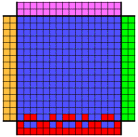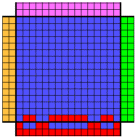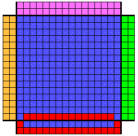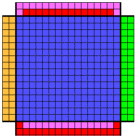1. Final edge set in this example has three complementary pairs of edge cubies that have a different sense to the other edge cubies in the set.
2. An appropriate sequence of rotations is applied to 1 to correct the problem for one of the complementary pairs (similar sequences are required to perform corrections on the remaining complementary pairs still requiring correction).
3. The sense of all the edge cubie elements in the final set is the same but just this one set needs to be flipped to resolve this problem (this can only occur for cubes of even size). Alternatively, action as indicated here can be avoided by flipping initially the inner complementary pair of edge cubies to match the correct final alignment and then matching all further complementary pairs of edge cubies to the initial pair.
4. The positions of two (and only two) edge cubie sets need to be swapped to resolve this problem (can occur only for cubes of even size). The solution to this problem can also be subdivided into a sequence of moves that applies to just four edge cubies at a time (as for the size 4 cube).

Items 1 to 3 refer to the OLL problem resolution and item 4 refers to the PLL problem resolution.

The correction of all of the parity problems mentioned above normally involves some re-arrangement of centre cubies (it can be shown that it is possible to resolve these problems for the size 4 cube without a rearrangement of centre cubies but not so for cubes of any larger size). Problems similar to those illustrated in images 1 and 2 occur in cubes of odd size. Rotation sequences required to resolve final layer problems for cubes of any size are similar to those required for the size 4 cube. The moves (algorithms) on which the graphics in this section are based are given in Instructions for Solving Cubes of Various Sizes. Those moves do not result in any change to any cubies except the relevant edge cubies and some centre cubies.

Because there are no distinguishing marks on the centre cubies when OLL and PLL corrections are made on standard cubes, it can be difficult to visualize what is happening. In the following illustrations the only cubies (centre and edge) that move position are shown in colour. Alphanumerical identification shows positional movement.

 OLL before correction for size 9 cube OLL after correction for size 9 cube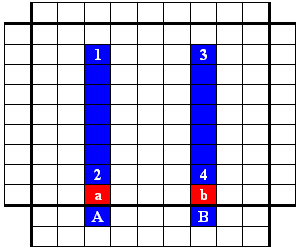For the OLL correction there are (n - 2) centre cubie swaps and overall there are (n - 1) swaps when the edge pair is included. For odd size cubes (n - 1) is always even (and conforms to the universal even parity requirement for odd size cubes). For even size cubes (n - 1) is always odd which means in this case a parity reversal always occurs, an allowable parity condition for even size cubes.

For the complete edge set flip (a requirement that can arise only for cubes of even size), the number of swaps will be (n - 1)(n/2 - 1). The overall number of swaps will be even if (n/2 - 1) is even (i.e. n/2 is odd). The overall number of swaps will be odd if n /2 is even. Hence overall parity will be even if n/2 is odd and odd if n/2 is even.

A typical PLL correction for a size 8 cube is illustrated below.

 PLL before correction for size 8 cube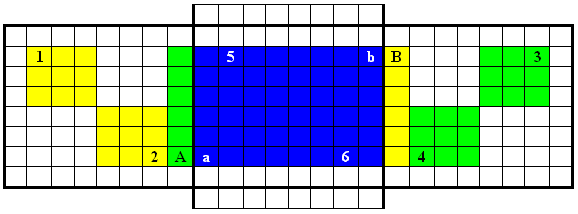PLL after correction for size 8 cube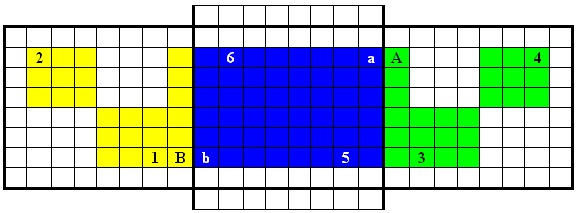For this PLL correction there are (n - 2)2 centre cubie swaps and since this correction is required only for cubes of even size, that value is always even. Likewise the number of edge cubies swaps (n - 2) is also even. Hence overall even parity applies for this permutation.

Initially consider the number of edge cubie flips required for a size n cube. If the centre pair for even size cubes or the middle edge cubie for odd size cubes, is left unchanged and all complementary pairs that don't match are flipped, then the maximum number of corrections (including the complete edge set flip as in image 3) that would be required for an even size cube is (n - 2)/2, and that for an odd size cube is (n - 3)/2. For large cubes, on average, one would expect about half the maximum number of swaps would be required (much like tossing a coin many times and observing that the heads count tends towards being the same as the tails count). For large cubes, one could expect that approximately n/4 edge cubie flip corrections would be required.

The PLL correction illustrated in image 4 is either not required or to be applied once for cubes of any even size greater than 3. Swapping any two edge sets (either opposite or adjacent) will resolve the edge-cubie-set position problem.

Any further adjustments required to the final layer for standard cubes, or for corner and edge cubies for cubes with marked centres, can be achieved using standard final layer algorithms for use with the size 3 cube. For cubes with marked centres special algorithms are required to achieve the alignment of centre cubies on the final face.

Back to Contents.

### 6.5 Algorithms

Instructions for people on how to solve Rubik's type cubes are normally conveyed either in purely graphical form or as sequences defined using a printable character notation. A character sequence that can be translated and applied to perform a sequence of layer rotations to transform a given state to another (usually less scrambled) state is often referred to as an algorithm. Algorithms are most commonly used when unscrambling the latter portion of the cube but can be applied more extensively if desired. Algorithms can be written down as instructions that can be memorized or looked up in a document. The printable characters used (e.g. to indicate an anticlockwise quarter turn, a single layer quarter turn or a multiple layer quarter turn) in algorithm instructions vary among authors as does their positions in the instructions. Where people interpret instructions the way they are presented is insignificant. The only time the form of presentation has significance is when computer keyboard entry is used to change the state of software cubes, and automatic updating of the screen image occurs whenever a valid instruction is received. For example if F' is used to represent an anticlockwise quarter turn of the front face then, as the user types in F, a clockwise quarter turn will occur and a correction will be needed when the user types the ' character. The end result will still be correct but use of -F rather than F' would eliminate the superfluous rotation. Any text enhancements, such as superscripts or subscripts, must be avoided in the method of presenting cube rotation sequences when users communicate with software cubes via keyboard commands. When computer keyboard entry of instructions is used, macros (which map a short input text string to a longer string) can be used as algorithm shortcuts.

Back to Contents.

### 6.6 Speedcubing

Speedcubing (or speedsolving) is the practice of trying to solve a cube in the Rubik cube family in the shortest time possible (which usually implies reducing the number of quarter turn moves required). It is most commonly applied to cubes of small size and there are numerous solving methods that have been documented. An international team of researchers using computer power from Google has found every way the standard size 3 Rubik's cube can be solved and have shown it is possible to complete the solution in 20 moves or less for any initial scrambled state. Generally, speed solving methods apply more to specialist cubists than typical cubists and are more complex than simple layer-by-layer type methods used by most other people.

## 7. Reachable and unreachable states for cubes of all sizes

If a cube has at some previous time occupied the set state, then any state that can arise after legal moves is considered to be a reachable state. For small size cubes (size 2, 3 or 4) an unreachable state is one that cannot be reached by legal moves. For larger cubes there needs to be some further qualification on what we intend to define as an unreachable state. In this article we exclude notional movement between 24-cubie orbits for edge and for centre cubies.

### 7.1 Relationship between reachable and unreachable states

If, for a cube of any size, r represents the number of reachable states, u represents the number of unreachable states and t equals their sum:

t = u + r
t = kr where k is an integer
u = (k - 1)r

Both r and k are functions of cube size n. Values for r and k will be considered in the following sections.

Back to Contents.

### 7.2 Reachable states for cubes of all sizes

The number of different states that are reachable for cubes of any size can be simply related to the numbers that are applicable to the size 3 and size 4 cubes. Hofstadter in his 1981 paper provided a full derivation of the number of states for the standard size 3 Rubik's cube. More recent information sources for the size 3 and size 4 cubes are also available. References that indicate the number of possible states for a size n cube are available. The brief material provided below presents the results in the form used in one of these references which covers the topic in far more detail.

For cubes with unmarked centre cubies the following integer constants (represented by P, Q, R and S) apply. These constants are in agreement with figures frequently quoted for the size 3 and size 4 cubes.

 Corner cubie possibilities for even size cubes P (7!) 36 3.67416000000000 x 106 Central edge cubie possibilities for odd size cubes, multiplied by 24 Q 24 (12!) 210 1.17719433216000 x 1013 Edge cubie possibilities for each dual set (12 pairs) R 24! 6.20448401733239 x 1023 Centre cubie possibilities for each quadruple set (6 groups of 4) S (24!)/(4!)6 3.24667053711000 x 1015

The value of S may warrant a word of explanation as it is commonly stated that the number of possible states for centre cubies with identifying markings for a size 4 cube is 24!. However, for each possible arrangement of edge cubies, only half those states are reachable. If the identifying markings are removed, we need to reduce the above number of possibilities by a factor of 4! for each set of four identical centre cubies, except for the last set of four which is halved to account for unreachable but unobservable states similar to those which applied for marked centre cubies. Because there are many ways of arranging the centre cubies that look the same there is no restriction on the observable arrangements of centre cubies in any given orbit. For example, if two red centre cubies are swapped no change in observable state results, but such an action without changing anything else would defy parity rules. If the unobservable unreachable states are ignored the correct answer still results but an incorrect answer will result for the number of possible states when centre cubies have identifying markings.

The number of possible states m for a size n cube can be defined in terms of the factors P, Q, R and S.

m = P Qa Rb Sc

where a, b and c are integer variables (functions of cube size n) as given below.

a  =  n mod 2 (i.e. 0 if n is even or 1 if n is odd)
b  =  0.5 (n - 2 -a)
c  =  0.25 ((n - 2)2 - a)

For even size cubes Qa = 1.

For further simplification, parameter m may also be expressed as m = 10 y where y = log10m. Parameter y can be related to n by a continuous quadratic function subject to the restriction that n must be an integer greater than 1 when referring to possible states for cubes:

y = An2 + Bn + C

where A, B and C are constants. Constants A and B are the same for n even and for n odd but the value of C is different.

 Parameter Value A 3.87785955497335 B -3.61508538481188 CEVEN -1.71610938550614 CODD -4.41947361312695 CEVEN - CODD 2.70336422762081

In graphical terms, when y is plotted, two parabolae of exactly the same shape are involved, with "even" cube values lying on one and "odd" cube values lying on the other. The difference is imperceptible except when plotted over a small range of n. as indicated in the graphs reproduced below. Only Rubik's family values for n equal to 2 or 3 are included in the second graph.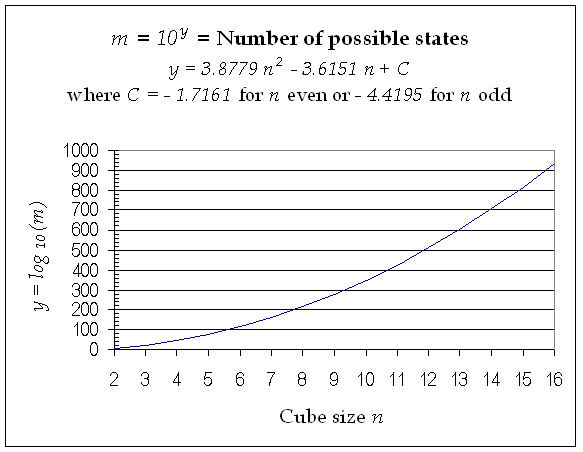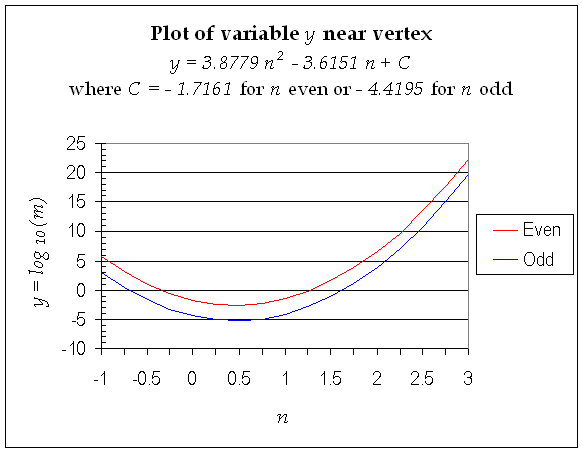Use of the log function y provides the only practical means of plotting numbers that vary over such a huge range as that for the Rubik's cube family. The difference between the curves translates as a factor of 505.08471690483. This is the factor that defines the effect of even size, relative to odd size, on the number of reachable states.

Hence, with the logarithmic presentation the number of cube states can be expressed using just four non-integer numbers (A, B and the two C values). Furthermore, the number of cube states form a restricted set of values for a more general continuous quadratic (parabolic) function for which n can have non-integer and negative values. Calculating the value of m from the corresponding value of y is a straight forward process.

Centre cubies are different from corner or edge cubies in that, unless they have indicative markings, there are multiple possibilities for their final orientation and/or locations. The number of different ways centre cubies can be arranged to yield a solved cube with unmarked centre cubies may be of interest. To calculate that, the impact of centre cubie marking needs to be assessed. Define mM, QM and SM to be the changed parameters for marked centre cubies (P and R remain unchanged).

QM  =  T Q   where T = 46/2 = 2048
SM  =  V S   where V = (4!)6/2 = 95551488
mM  =  P (QM)a Rb (SM)c
mM  =  mD m
mD  =  Ta Vc

Parameter mM defines the number of reachable states for cubes with marked centres. Factor mD gives the number of different arrangements of unmarked centre cubies that will provide a solved size n cube. It is also the factor by which the number of different states for a standard cube needs to be multiplied by when marked centres apply.

Back to Contents.

### 7.3 Unreachable states for cubes of all sizes

The number of unreachable states far exceeds the number of reachable states. There are many references to the number of unreachable states for the size 3 cube but very few for larger size cubes.

The unreachable arrangements for corner and edge cubies are the same for cubes with or without marked centres.

If we consider a corner cubie for cubes of any size then a 1/3 twist clockwise leaving everything else unchanged will represent an unreachable state, and similarly for a 1/3 twist counter-clockwise. Hence only 1/3 of the twist possibilities are reachable.

For the central edge cubie for odd size cubes the behaviour is the same as that for the size 3 cube. Only half the conceivable positions are reachable and only half the conceivable orientations are reachable. Hence only 1/4 of the central edge cubie movement possibilities are reachable.

For the edge cubie sets, comprising 12 complementary pairs (24 cubies total), there is no restriction on position as for the central edge cubies for odd size cubes. In simple terms, an arrangement arrived at by flipping any one edge cubie in the set without changing something else represents an unreachable state. This implies that half the arrangements are reachable. Alternatively, the possibilities for any complementary pair can be considered.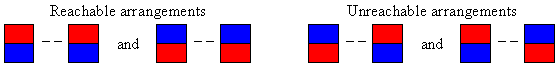In effect, half the conceivable arrangements are reachable. If both of the companion edge cubies, irrespective of where they are located, are flipped a reachable arrangement results. If two non-matching edge cubies are flipped, their effect is non-cancelling with two matching pairs now having a quarter of their conceivable arrangements being unreachable. Once again the two quarters added together represents half the conceivable states being unreachable.

In the case of the marked centre cubies only half the conceivable arrangements for each set of 24 cubies for any given orbit are reachable. The same parity rules that apply for marked centre cubies also apply for the unmarked centre cubies. A quarter turn of a set-of-four centre cubies cannot be achieved without changing the arrangement elsewhere to meet the parity requirement. Because there are 95551488 ways of arranging the individual centre cubies so that the resulting arrangement appears exactly the same, parity rules can be met without any observable indication of how the parity compliance is achieved. Hence, for the normal case (24 cubies comprising four of each of six colours) there is no restriction on the achievable states for the centre cubies.

The following table uses the values noted above to represent the k component factors for the size n cube. Exponents a, b and c are functions of cube size n as defined above.

 Reduction components for factor k (for standard cube with unmarked centres) and for kM (for cube with marked centres) Unmarked centres' cube type Marked centres' cube type Corner cubie factor 3 3 Central edge cubie factor (exists only for cubes of odd size) 22a 22a Complementary edge cubie factor for all 12-pair sets combined 2b 2b Absolute centre cubie factor for odd size cubes 1 2a Centre cubie factor for all 24-cubie sets combined 1 2c

Taking the product of these factors:

 For the standard size n cube k = (3)22a + b For the marked centres' size n cube kM = (3)23a + b + c

Some values for cubes of small size are given below.

 Cube size 2 3 4 5 6 7 8 Value of k 3 12 6 24 12 48 24 Value of kM 3 24 12 192 192 6144 12288

The number of unreachable states is given by (k - 1)m for standard cubes and by (kM - 1)mM for cubes with marked centre cubies.

Back to Contents.

## References

Back to Contents.